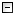Home Control Panel Login Register Search Submit Logout About me Contact meMy ResearchWeb This Site
 Home > How to use web statistical analysis
How to use web statistical analysis
Cite this page: (2008). How to use web statistical analysis. Retrieved December 4, 2023, from https://www.psychstat.org/us/article.php/76.htm.
How to use web statistical analysis (WebStatR)

This is a short document on how to use Web Statistical Analysis in R (WebStatR) tools.

0. Interface of WebStatR.

1. Basic analysis

Essentially, any R codes that can run on your desktop can be implemented in WebStatR. For example, you can copy and paste the following R codes into the code window,

x<-rnorm(100,5,2)
y<-10 + 5*x + rnorm(100)
reg<-lm(y~x)
summary(reg)

In the results, you will get

## Results

> x<-rnorm(100,5,2)
> y<-10 + 5*x + rnorm(100)
> reg<-lm(y~x)
> summary(reg)

Call:
lm(formula = y ~ x)

Residuals:
Min 1Q Median 3Q Max
-2.60479 -0.55080 -0.05718 0.52724 2.65388

Coefficients:
Estimate Std. Error t value Pr(>|t|)
(Intercept) 9.94837 0.24187 41.13 <2e-16 ***
x 5.02443 0.04283 117.31 <2e-16 ***
---
Signif. codes: 0 '***' 0.001 '**' 0.01 '*' 0.05 '.' 0.1 ' ' 1

Residual standard error: 0.9038 on 98 degrees of freedom
Multiple R-squared: 0.9929, Adjusted R-squared: 0.9929
F-statistic: 1.376e+04 on 1 and 98 DF, p-value: < 2.2e-16

2. Plot

WebStatR supports generating figures using R graphic functions, such as plot(). For example, the following codes will generate a histogram:

y<-rnorm(100)
hist(y)

Another example:

plot(cars, main="Stopping Distance versus Speed")
lines(lowess(cars))
text(10,100,"This is an example",col='red')

Userd can upload their own data sets for analysis. To upload the data file, click on the "Brownse..." button and choose the file to the data file to use. Please note that only .txt and .dat file can be used.  The data should be in free format, only space to seperate the data. The uploaded data set will be read by the function read.data() and the data are then stored in a data frame called "userdata". The variables names are not allowed in the data file.

mean(userdata\$V1) calculates the mean of the first variable in the data. hist(userdata\$V1) gives the histogram.

4. Utilities

Some utilites can be used to make the data analysis easier.

5. Functions that are not allowed to use

Submitted by: johnny
 Add a comment View comment Add to my favorite Email to a friend Print1 2 3 4 5 6 7 8 9 10
 Copyright © 2003-13 Zhiyong Zhang \'s Psychometric WebsiteMaintained by Zhiyong Zhang